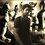# Derivation needed

$if\quad { x }^{ 2 }=x+1\quad and\quad n\ge 2\quad Then\quad { x }^{ n }={ f }_{ n }x+{ f }_{ n-1 }$

Can anybody provide me a derivation for this Lemma ? I was able to prove this lemma by applying the principle of mathematical induction. However I believe there are variety of proofs and derivations for this lemma. It would be better if all the proofs and derivations are posted in this note. A BIG THANKS to contributors.Note by Inderjeet Nair
6 years, 3 months ago

This discussion board is a place to discuss our Daily Challenges and the math and science related to those challenges. Explanations are more than just a solution — they should explain the steps and thinking strategies that you used to obtain the solution. Comments should further the discussion of math and science.

When posting on Brilliant:

• Use the emojis to react to an explanation, whether you're congratulating a job well done , or just really confused .
• Ask specific questions about the challenge or the steps in somebody's explanation. Well-posed questions can add a lot to the discussion, but posting "I don't understand!" doesn't help anyone.
• Try to contribute something new to the discussion, whether it is an extension, generalization or other idea related to the challenge.
• Stay on topic — we're all here to learn more about math and science, not to hear about your favorite get-rich-quick scheme or current world events.

MarkdownAppears as
*italics* or _italics_ italics
**bold** or __bold__ bold
- bulleted- list
• bulleted
• list
1. numbered2. list
1. numbered
2. list
Note: you must add a full line of space before and after lists for them to show up correctly
paragraph 1paragraph 2

paragraph 1

paragraph 2

[example link](https://brilliant.org)example link
> This is a quote
This is a quote
    # I indented these lines
# 4 spaces, and now they show
# up as a code block.

print "hello world"
# I indented these lines
# 4 spaces, and now they show
# up as a code block.

print "hello world"
MathAppears as
Remember to wrap math in $$ ... $$ or $ ... $ to ensure proper formatting.
2 \times 3 $2 \times 3$
2^{34} $2^{34}$
a_{i-1} $a_{i-1}$
\frac{2}{3} $\frac{2}{3}$
\sqrt{2} $\sqrt{2}$
\sum_{i=1}^3 $\sum_{i=1}^3$
\sin \theta $\sin \theta$
\boxed{123} $\boxed{123}$

## Comments

Sort by:

Top Newest

Well, use Binet's Formula. You will get it. It's easy. Binet's formula is ${f}_{k} = \frac{{\phi}^{k} - {(1-\phi)}^{k}}{\sqrt{5}}$

- 6 years, 3 months ago

Log in to reply

I presume Binet's formula is derived from this lemma. Today when I asked my cousin about the derivation, he gave a resolved derivation which is understandable for almost everyone. First of all in this lemma GOLDEN RATIO is already given.

Golden ratio:${ x }^{ 2 }=x+1$

It is also given that $n\ge 2$

Now,$\quad { x }^{ 3 }={ x }^{ 2 }.x\\ \therefore \quad { x }^{ 3 }=(x+1).x\quad ....{ (x }^{ 2 }=x+1)\\ \therefore \quad { x }^{ 3 }={ x }^{ 2 }+x\\ \therefore \quad { x }^{ 3 }=2x+1\quad ....{ (x }^{ 2 }=x+1)$

Similarly it can be proven that,

${ x }^{ 4 }=3x+2\\ { x }^{ 5 }=5x+3\\ { x }^{ 6 }=8x+5$

Thus we can conclude that ${ x }^{ n }={ f }_{ n }x+{ f }_{ n-1 }$

We know the fact that the roots of golden ratio are $\phi$ and $1-\phi$

Now we can deduce two equations:

${ \phi }^{ n }=f_{ n }\phi +{ f }_{ n-1 }\quad \quad \quad \quad \quad \quad \quad \quad ....1)\\ (1-{ \phi ) }^{ n }=f_{ n }(1-\phi )+{ f }_{ n-1 }\quad \quad ....2)$

Subtract equation 2 from 1 to yield Binet's formula

This was taught to me by my cousin. So, from above, I think Binet's formula was derived from this lemma.

- 6 years, 3 months ago

Log in to reply

Yeah I thought of that too but I thought Binet's formula would have been better.

- 6 years, 3 months ago

Log in to reply

Can you please explain in detail on how we can use this formula to derive the above Lemma?

- 6 years, 3 months ago

Log in to reply

Yes you can easily prove using binets formula

To prove the binets formula, i will give a hint

the nth fibonnaci ($f_n$ ) number is the coefficient of $x^n$ in

$\frac {1}{1-x-x^2}$

now use partial fraction to break it down, you will know your answer as soon as you will do that

btw $\phi \quad$ is one of the roots of the denominator of the infinite polynomial i gave

(note that the denominator is not the same quadratic as the one you gave, but the roots of this one and the one you gave are very common, you will see)

- 6 years, 3 months ago

Log in to reply

Can you please elaborate.

- 6 years, 3 months ago

Log in to reply

can you please tell what $f_n$ stands for?

- 6 years, 3 months ago

Log in to reply

Fn is the nth fibonacci number

- 6 years, 3 months ago

Log in to reply

×

Problem Loading...

Note Loading...

Set Loading...